• pandas删除行值为0
千次阅读
2020-10-12 09:31:06

删除某行值为0

先求出值为0所在行的索引

然后根据索引行删除

df.drop( index = df.age[df1.age == 0].index )python 索引
更多相关内容
• 1.删除/选取某列含有特殊数值的 import pandas as pd import numpy as np a=np.array([[1,2,3],[4,5,6],[7,8,9]]) df1=pd.DataFrame(a,index=['row0','row1','row2'],columns=list('ABC')) print(df1) df2=df1....
• 以上所述是小编给大家介绍的pandas删除指定详解整合，希望对大家有所帮助，如果大家有任何疑问请给我留言，小编会及时回复大家的。在此也非常感谢大家对软件开发网网站的支持！ 您可能感兴趣的文章:Pandas过
• 使用pandas处理数据时，如何实现删除/选取某列含有特殊数值的或者某含有特殊数值的列，以及如何去除含有空值的或列
• 首先应该判断自己表格中的None的类型 表格中None有两种情况： （1）空值类型的None。 （2）字符串类型的None，是真实存在的。 处理空值类型的None ...df.dropna(how='all')#删除所有内容均缺失 df.drop

### 首先应该判断自己表格中的None的类型

表格中None有两种情况：

（1）空值类型的None。

（2）字符串类型的None，是真实存在的。

### 处理空值类型的None

df.dropna()方法

#axis=0: 删除包含缺失值（NaN）的行

#axis=1: 删除包含缺失值（NaN）的列

# how=‘any’ :要有缺失值（NaN）出现删除

# how=‘all’: 所有的值都缺失（NaN）才删除

df.dropna(how='all')#删除所有内容均为缺失值的行
df.dropna(axis=1) #丢弃有缺失值的列
df.dropna(axis=1, how = 'all') #丢弃所有列中所有值均缺失的列
df.dropna(axis=0, subset=['datetime', 'values'])#丢弃datetime和values这两列中有缺失值的行

### 处理字符串类型的None

可以先将“None”值replace为pandas可读取的空值，如nan，然后再用dropna()去掉即可。

df = df.replace(to_replace='None', value=np.nan).dropna()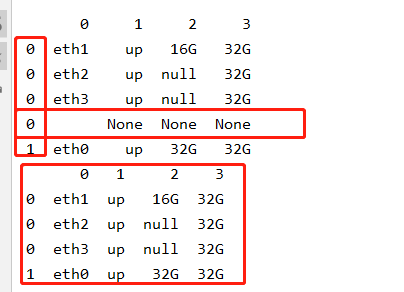参考链接 原文链接：https://blog.csdn.net/Shenquanle/article/details/115319674

展开全文python
• 使用pandas对数据操作，筛选数据时，根据任务要求有时不仅要某列中存在空值的，并且要删除某列中指定所在。 1.data.dropna() 默认参数： data.dropna(axis=0, how='any', thresh=None, subset=None, in...

使用pandas对数据操作，筛选数据时，根据任务要求有时不仅要某列中存在空值的行，并且要删除某列中指定值所在行。

## 1.data.dropna()

默认参数：
data.dropna(axis=0, how='any', thresh=None, subset=None, inplace=False)

### 1-1 axis确定删除存在缺失值的行或者是列

#删除含有缺失值的行
axis=0或axis='index’

#删除含有缺失值的列
axis=1或axis='columns’

### 1-2 how 确定存在缺失值时，是否删除行或者列

how='all’或how=‘any’。

how='all’时表示删除全是缺失值的行（列）

how='any’时表示删除只要含有缺失值的行（列）

### 1-3 thresh=n表示保留至少含有n个非na数值的行

data.dropna(thresh=2)

### 1-4 subset确定要在哪些列中查找缺失值

#在source和target两列中查找缺失值
data.drop(subset = ["source","target"])

### 1-5 inplace确定是否直接在原DataFrame修改

#删除缺失值后不在原data上修改
inplace = False
#删除缺失值后在原data上修改
inplace = True

## 2.data.drop

默认参数：
data.drop(
labels=None,
axis=0,
index=None,
columns=None,
level=None,
inplace=False,
errors='raise',
)

### 2-1 labels 指定行或者列的名称

#参数axis为0表示在0轴（列）上搜索名为“姓名”的对象，然后删除对象“姓名”对应的行。
data.drop("姓名",axis = 0)

#参数axis为0表示在1轴（行）上搜索名为“姓名”的对象，然后删除对象“姓名”对应的列。
data.drop("姓名"，axis = 1)

### 2-2 index 指定要删除的行

#删除data中索引为0和1的行
data.drop(index = [0,1])

### 2-3 columns 指定要删除的列

#删除data中列名为“source”和“target”的列
data.drop(columns=['source', 'target'])

## 3.实例

任务需求：删掉“ZH_Term_len”列中值为0的全部行。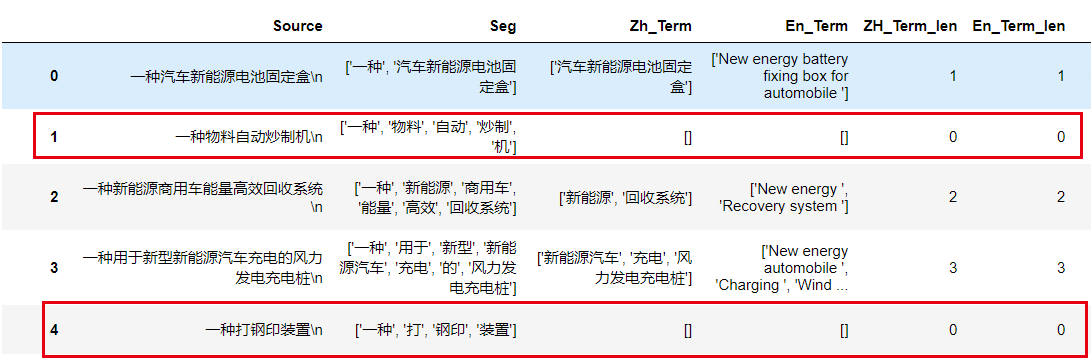### 3-1 统计0的数量

#统计“ZH_Term_len”一列中有多少个0
data["ZH_Term_len"].value_counts()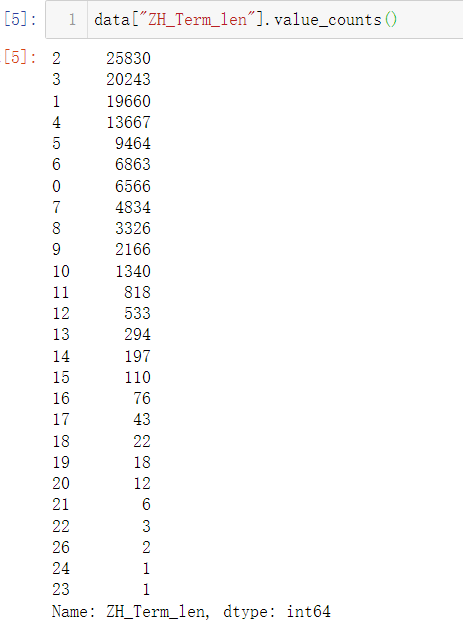### 3-2 找出0的索引

data[(data.ZH_Term_len == 0)].index.tolist()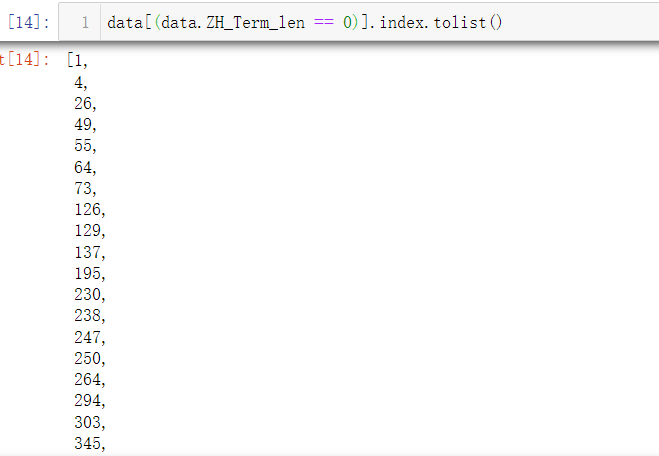### 3-3 使用drop函数以及index参数删除所在的行

data =  data.drop(index = data[(data.ZH_Term_len == 0)].index.tolist())

### 3-4 查看数据

data.info()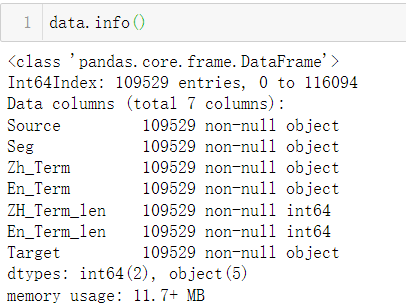### 3-5 将索引重新排序

#会将标签重新从零开始顺序排序,使用参数设置drop=True删除旧的索引序列
data = data.reset_index(drop=True)

### 3-6 统计“ZH_Term_len”列中值的数量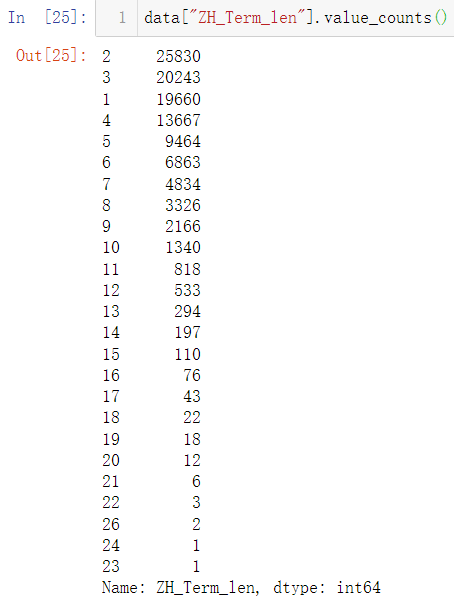统计后发现，“ZH_Term_len”列中值为0的行已经全部被删除掉。

展开全文python
• 主要介绍了pandas.DataFrame中删除包涵特定字符串所在的，文中通过示例代码介绍的非常详细，对大家的学习或者工作具有一定的参考学习价值，需要的朋友们下面随着小编来一起学习学习吧
• 要使用np.delete删除它们。 示例1 import numpy as np a = np.array([[1, 2, 0, 3, 0], [4, 5, 0, 6, 0], [7, 8, 0, 9, 0]]) idx = np.argwhere(np.all(a[..., :] == 0, axis=0)) a2 = np.delete(a, idx, axis=1...
• 数据集df中，对于属性appPlatform（最后一列），我们想删除掉取值2的那些样本。如何做？非常简单。 import pandas as pd df[(True-df['appPlatform'].isin())] 当然，有时候我们需要去掉不止一个，这个...
• Overview df.loc[:, (df != 0).any(axis=0)] Reference How do I delete a column that contains only zeros in Pandas?
• ## Overview

df.loc[:, (df != 0).any(axis=0)]

• ## Reference

1. How do I delete a column that contains only zeros in Pandas?
展开全文• 在数据分析中，处理缺失是数据清洗中的第一步。 如何找出并丢掉缺失所在？ 如何找出并丢掉缺省所在？？ 有没有图可以直观展示缺失？？？
• pandas删除dataframe中行内容全是0的数据（remove rows with all zeros in dataframe）python 数据挖掘 机器学习 人工智能
• cell_df = cell_df.dropna(axis=0, how='any')#删除任何包含nan的 删除pandas.DataFrame 中包含NaN的或列_Transfer-CSDN博客_pandas删除nan的机器学习 python 深度学习
• pandas初步删除明显错误的，对数据做初步清洗 def del_error_data(self, data): data = data[data.IAS.between(0, 70, inclusive=True)] data = data[data.Throttle.between(0, 115, inclusive=True)] data = ...python
• 运行环境：python3 作者：K同学啊 精选专栏：《深度学习100例》 推荐专栏：《新手入门深度学习》 极品专栏：《Matplotlib教程》 ...# axis参数默认为0，axis=0这个可以省略 df.drop([0, 1], axis=0) ...python
• 删除某列中值空的 先求出值为空所在的索引 然后根据索引删除 df.drop( df.age[df.age.isna()].index); 或者直接df.dropna()索引 python
• ## Python pandas删除指定行/列数据

万次阅读 多人点赞 2021-03-16 17:31:56
滤除缺失数据dropna()1)滤除含有NaN的所有2)滤除含有NaN的所有列3)滤除元素都是NaN4)滤除元素都是NaN的列5)滤除指定列中含有缺失的2.删除重复 drop_duplicates()3.根据指定条件删除行列drop() 1....python
• 1.相关函数 df.dropna() df.fillna() df.isnull() df.isna() 2.相关概念 空值：在pandas中的空值是”” ...axis:维度，axis=0表示index,axis=1表示columns列，默认为0 how:”all”表示这一
• 通过pandas删除列： 1.del df[‘columns’] ...删除不改表原始数据，可以通过重新赋值的方式赋值该数据，axis默认为0，用此函数可以删除 3.df.drop(‘columns’,axis=1,inplace=‘True’) 改变原始数据 ...机器学习 深度学习 python
• df.dropna(subset = [‘需要考虑空值的列’])
• 我想删除列名公交车站、地铁站、居住、工作、游憩、poi_s这几列同时零的，比如和第5514数据相似的有问必答 python
• 我已经在this线程中阅读了答案,但它没有回答我的确切问题.我的DataFrame看起来像这样Lady in the Water The Night Listener Just My Luck CorrelationClaudia Puig NaN 4.5 3.0 0.893405Gene Se...
• 4、将分数列值为NAN（空值）的填充为0分 5、将姓名的缺失进行填充 6、将清洗好的数据保存到指定excel中 原始数据： 最后输出数据： 处理步骤： 1、读取数据： 2、删除全是空值的列 3、删除全是空值的 4、将...
• ## pandas删除某一行/列

千次阅读 2021-11-01 11:40:31
pandas 删除某一/列 ...axis 默认为0，指删除行，因此删除columns时要指定axis=1； index 直接指定要删除 columns 直接指定要删除的列 inplace=False，默认该删除操作不改变原数据，而是返回一个python 开发语言 后端
• drop_duplicates（subset，keep，inplace，ignore_index） DataFrame.drop_duplicates(subset=None, keep='first', in...选择需要保留的重复，有三个选项， - first：保留第一次出现的； - last：保留最后一次出html
• 删除重复的index dataframe[~dataframe.index.duplicated(keep='first')] 根据某一列删除重复 dataframe.drop_duplicates(subset=['列1','列2',])...